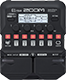# PPDST

Discussion in 'Zoom G1/G1X Four' started by Charmer, Jun 13, 2021.

1. PPDSTDevice: Zoom G1 Four
Firmware: 2.00

Name on device: PPDST
Optimized for: Guitar Amp

Effects chain:For passive pickups

Effect: "ZNR" (Dynamics), active - "yes"
"Detect" = EFXIN
"Depth" = 50
"Threshold" = 20
"Decay" = 10

Effect: "MetalWRLD" (Overdrive / Distortion), active - "yes"
"Gain" = 100
"Bass" = 81
"Treble" = 100
"Volume" = 90

Effect: "MK1 1x12" (Cabinet), active - "yes"
"MIC" = ON
"D57: D421" = 66
"Hi" = 70
"Lo" = 50

Effect: "Gt GEQ 7" (Filter), active - "yes"
"100" = -3.5
"200" = -3.0
"400" = -3.0
"800" = -6.0
"1.6k" = -4.0
"3.2k" = -4.0
"6.4k" = -2.0
"VOL" = 100

Effect: "ParaEQ" (Filter), active - "yes"
"Frequency" = 20.0kHz
"Q" = 4.0
"Gain" = 12.0
"Volume" = 82

Patch Volume: 41